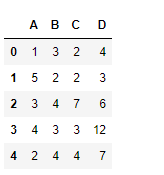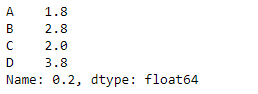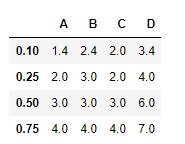Python | Pandas dataframe.quantile()

• Difficulty Level : Hard
• Last Updated : 22 Nov, 2018

Python is a great language for doing data analysis, primarily because of the fantastic ecosystem of data-centric python packages. Pandas is one of those packages and makes importing and analyzing data much easier.

Pandas dataframe.quantile() function return values at the given quantile over requested axis, a numpy.percentile.

Note : In each of any set of values of a variate which divide a frequency distribution into equal groups, each containing the same fraction of the total population.

Syntax: DataFrame.quantile(q=0.5, axis=0, numeric_only=True, interpolation=’linear’)

Parameters :
q : float or array-like, default 0.5 (50% quantile). 0 <= q <= 1, the quantile(s) to compute
axis : [{0, 1, ‘index’, ‘columns’} (default 0)] 0 or ‘index’ for row-wise, 1 or ‘columns’ for column-wise
numeric_only : If False, the quantile of datetime and timedelta data will be computed as well
interpolatoin : {‘linear’, ‘lower’, ‘higher’, ‘midpoint’, ‘nearest’}

Returns : quantiles : Series or DataFrame
-> If q is an array, a DataFrame will be returned where the index is q, the columns are the columns of self, and the values are the quantiles.
-> If q is a float, a Series will be returned where the index is the columns of self and the values are the quantiles.

Example #1: Use quantile() function to find the value of “.2” quantile

 # importing pandas as pdimport pandas as pd  # Creating the dataframe df = pd.DataFrame({"A":[1, 5, 3, 4, 2],                   "B":[3, 2, 4, 3, 4],                   "C":[2, 2, 7, 3, 4],                    "D":[4, 3, 6, 12, 7]})  # Print the dataframedfLet’s use the dataframe.quantile() function to find the quantile of ‘.2’ for each column in the dataframe

 # find the product over the index axisdf.quantile(.2, axis = 0)

Output :Example #2: Use quantile() function to find the (.1, .25, .5, .75) qunatiles along the index axis.

 # importing pandas as pdimport pandas as pd  # Creating the dataframe df = pd.DataFrame({"A":[1, 5, 3, 4, 2],                   "B":[3, 2, 4, 3, 4],                   "C":[2, 2, 7, 3, 4],                   "D":[4, 3, 6, 12, 7]})  # using quantile() function to# find the quantiles over the index axisdf.quantile([.1, .25, .5, .75], axis = 0)

Output :My Personal Notes arrow_drop_up